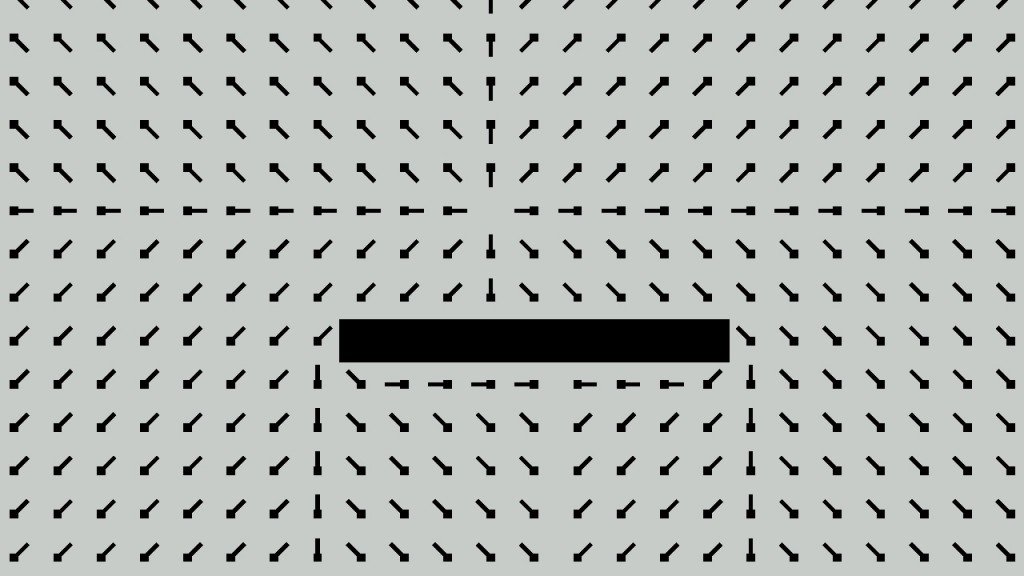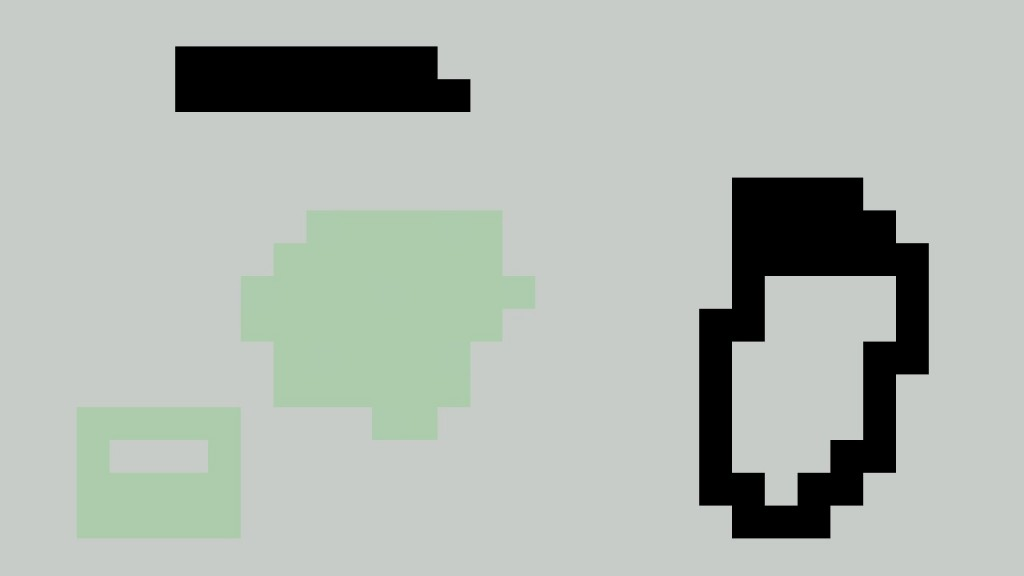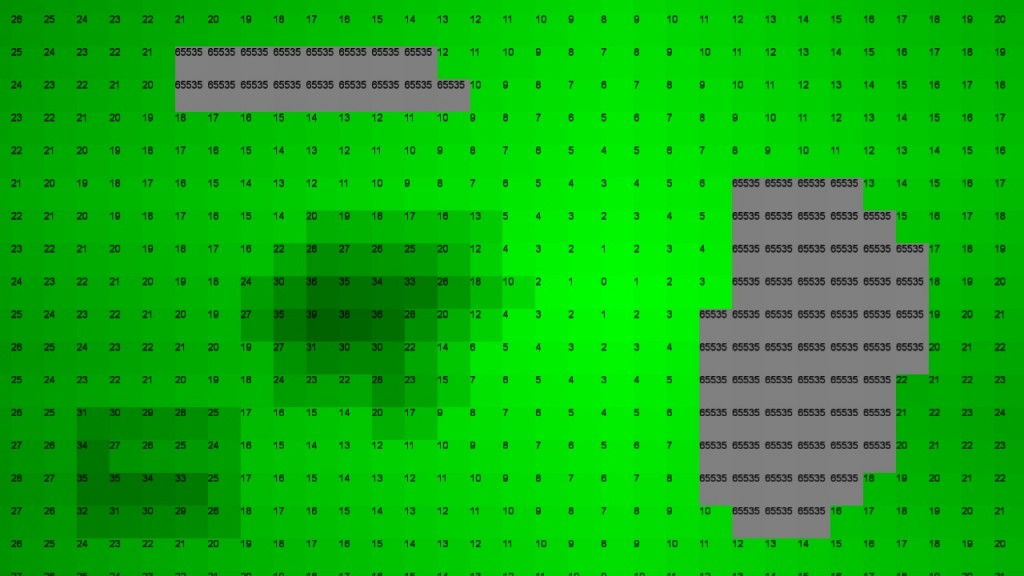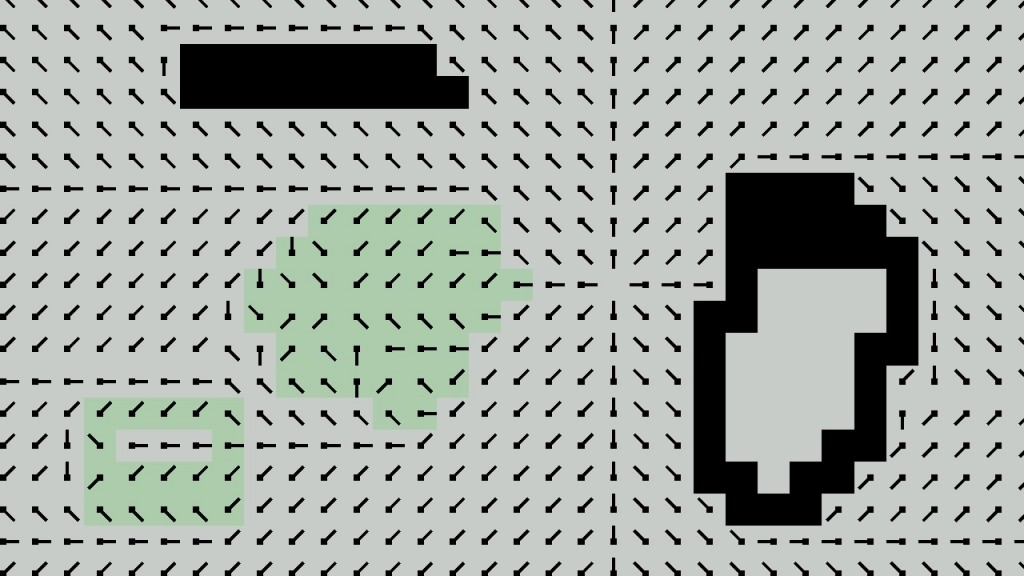# Flow Field Pathfinding

One of the most important and wide reaching subjects of artificial intelligence in video games is pathfinding. One of the most commonly used methods of pathfinding in games at the moment is the A* algorithm. This algorithm is capable of finding the shortest path between two points when given a graph to traverse. This method is an acceptable solution to pathfinding in most situations, but there are a few cases where A* is not able to meet the requirements of an application. When an application fulfills one or more of these cases, flow field pathfinding may become an attractive solution.

Some of these cases where regular A* may not be the best choice are:

• If the graph that needs to be traversed is dense (A common example would be a grid-based graph)
• If you have hundreds of units attempting to path to the same location
• If the environment is highly dynamic
• If there is a constant need to change the position of units

There are solutions to all of these issues using common techniques.

If the graph is dense, then allow a pathfinder to calculate paths in a non-blocking manner such that each unit sends a request to the pathfinder and idles until the pathfinder completes the request.

If you have hundreds of units attempting to reach the same location, then you can use Dijkstra’s algorithm to solve for all shortest paths from the goal to every other point on the graph, and then reconstruct paths for each unit using the same results. Flow field pathfinding uses an extension of this technique.

If the environment is dynamic, then an implementation of D* can be used. D* goes out and recalculates only the portions of a graph that are affected by changes.

If units need to be affected by forces other than the path that they are following, then their paths may need to be rebuilt or modified whenever a change occurs. This can be fixed by using Navigation Meshes which allow for changes in the position of units within a threshold before the path needs to be rebuilt.

The major issue is that each of these techniques can be time consuming and complicated to implement and get working together.

## Flow Fields

The first place that I heard about flow fields was through a live stream about the development of the game Planetary Annihilation. This article is a more simplified implementation of the one described by Elijah Emerson in his article about crowd pathfinding which goes into more detail about building on this algorithm to efficiently scale to large maps. I have listed the article in the sources list at the bottom of this page. The concept of flow fields has only started to come into use in computer games over the past few years. Before computer games, they were mostly used in fluid dynamics simulations as the name “flow field” suggests. Flow fields can also be referred to as vector fields.In vector fields each vector points towards their neighbor node closest to the goal. When a unit passes over a cell, the unit queries the vector that the node has. This vector is then used to influence the final velocity of the unit. True to the name the vectors in the flow field can look like the flow of water going around obstacles.

## Calculating the Flow Field

There are three fields that are used in the process of calculating the flow field. The first field is the cost field which stores the cost information associated with the terrain. The second is the integration field which is what does most of the work in calculating the field. The final field is the flow field itself which uses the integration field as input to calculate a final result.

Calculating a flow field involves three stages:

1. Create a cost field
2. Generate an integration field
3. Generate the flow field

#### The Cost FieldEach cell in the cost field is represented by a single byte that will normally be set to some value in between 1 and 255. By default all cells are set to a value of 1. Any values between 2 and 254 represent cells that are passable but should be avoided if possible. The value 255 represents impassable walls that units must path around.

Whenever the cost field is modified the integration fiend and integration field must be recalculated. Since units only read from the flow field and are not given static paths the whole recalculation process takes a constant amount of time regardless of the number of units using the field.

#### The Integration FieldThe integration field is where most of the work in the flow field calculation is done. In order to create the integration field we will use a modified version of Dijkstra’s algorithm.

This is a list of the steps that the algorithm takes to calculate the field:

1. The algorithm starts by resetting the value of all cells to a large value (I use 65535).
2. The goal node then gets its total path cost set to zero and gets added to the open list. From this point the goal node is treated like a normal node.
3. The current node is made equal to the node at the beginning of the open list and gets removed from the list.
4. All of the current node’s neighbors get their total cost set to the current node’s cost plus their cost read from the cost field then they get added to the back of the open list. This happens if and only if the new calculated cost is lower than the old cost. If the neighbor has a cost of 255 then it gets ignored completely.
5. This algorithm continues until the open list is empty.

In addition to being used for calculating the flow field, the integration field can also be used for reachability analysis. Since the algorithm will be blocked by impassable terrain, if a unit is on a cell of the integration field that has a value of 65535 then it can know that it will not be able to reach the goal unless the cost field changes.

Example implementation of the algorithm

void IntegrationField::calculateIntegrationField(unsigned targetX, unsigned targetY)
{
unsigned int targetID = targetY * mArrayWidth + targetX;

resetField();//Set total cost in all cells to 65535
list openList;

//Set goal node cost to 0 and add it to the open list
setValueAt(targetID, 0);
openList.push_back(targetID);

while (openList.size() > 0)
{
//Get the next node in the open list
unsigned currentID = openList.front();
openList.pop_front();

unsigned short currentX = currentID % mArrayWidth;
unsigned short currentY = currentID / mArrayWidth;

//Get the N, E, S, and W neighbors of the current node
std::vector neighbors = getNeighbors(currentX, currentY);
int neighborCount = neighbors.size();

//Iterate through the neighbors of the current node
for (int i = 0; i < neighborCount; i++)         {             //Calculate the new cost of the neighbor node             // based on the cost of the current node and the weight of the next node             unsigned int endNodeCost = getValueByIndex(currentID)                          + getCostField()->getCostByIndex(neighbors[i]);

//If a shorter path has been found, add the node into the open list
if (endNodeCost < getValueByIndex(neighbors[i]))
{
//Check if the neighbor cell is already in the list.
//If it is not then add it to the end of the list.
if (!checkIfContains(neighbors[i], openList))
{
openList.push_back(neighbors[i]);
}

//Set the new cost of the neighbor node.
setValueAt(neighbors[i], endNodeCost);
}
}
}
}

#### The Flow FieldFinally the flow field takes the result of the integration field’s calculations and uses it to determine the direction of the vectors in the field. The flow field calculates this by going through each cell of the integration field and comparing that cell’s value to all of its 8 neighbors to find the one with the lowest value. In this implementation I use a static array of 9 vectors (8 for directions and one for a zero vector) and then store the index of the vector that points towards to lowest neighbor in a 1 byte field. If the current cell is unreachable then the index is set to the zero vector.

## Performance

Here are some of my quick benchmarks on the time to calculate a grid.

• 10×10 : 0.2ms
• 20×20 : 1.0ms
• 25×25 : 1.5ms
• 40×40 : 3.7ms
• 50×50 : 5.2ms
• 100×100 : 20ms

This is compiled in C++ and uses the algorithm that I demonstrated earlier. Note that these results are based on a completely empty cost field. The time can increase by around 30% depending on how the map is laid out. However, it generally runs faster if there are more walls. These tests were run on release build. In debug builds calculation of the fields would generally take up to 15 times longer.

This is efficient enough for use on my computer, but slower computer would certainly struggle with 50×50 size fields and up. Treating each cell as 1m x 1m allows me to have plenty of space on the map for my units, however in larger games that need to cover several square kilometers it would be much more efficient to partition the fields. For instance instead of using a 100×100 field I could use 100 10×10 fields.

Having multiple fields adds a degree of difficulty, but can be done with very few changes to existing code. A method for doing pathfinding like this is described in the flow field article in the book Game AI Pro written by Elijah Emerson. What you end up doing is connecting the 10×10 fields with portals that span the gap between 1 to all of the nodes on an edge of the field. You then use A* to find a path from the current field to the target field and only calculate fields for each field that you need to pass through. Results from the A* and flow field calculation can be reused between multiple units. With this method maps can be far larger without performance issues.

## Following the Path

Now in order to make the units follow the flow field you just need to translate the position of the unit into the coordinate system of the flow field and get the corresponding vector to their cell. In my sample this is done by just dividing the unit’s position by the scale of the grid cells. I then take the new position and truncate it into integer x and y coordinates are then used to get the index of the vector stored in the cell that the unit occupies.

## Float-based Fields

My examples used mostly integers to store the cost, integration, and flow fields. This is due to the small space and efficiency gains that are involved. It is perfectly valid to use floats for all three fields. Using float-based cost and integration fields allows for much more fine control over the map and pathfinding. If you store 2D vectors in the flow field then much smoother movement is possible than when units are constrained to 8 discrete directions.

## Conclusion

Using flow fields in pathfinding allows for many advantages above other pathfinding techniques in some cases and allows for much more robust path following.

Comment if you have any questions.

### References

Durant, S. (2013, July 5). Understanding Goal-Based Vector Field Pathfinding. Tuts+. Retrieved December 05, 2013, from http://gamedevelopment.tutsplus.com/tutorials/goal-based-vector-field-pathfinding–gamedev-9007

Emerson, E. (2013). Crowd Pathfinding and Steering Using Flow Field Tiles. In S. Rabin (Ed.), Game AI Pro: Collected Wisdom of Game AI Professionals (pp. 307-316). Boca Raton: CRC Press.

Pentheny, G. (2013). Efficient Crowd Simulation for Mobile Games. In S. Rabin (Ed.), Game AI Pro: Collected Wisdom of Game AI Professionals (pp. 317-323). Boca Raton: CRC Press.

11-07520010-143484
01-3142
09-083136
11-071672
09-29155
03-172480
10-111984
02-022072
07-17977
04-132万+
05-211393
08-188852
07-201694
07-28374
09-211797
07-012803
©️2020 CSDN 皮肤主题: 大白 设计师:CSDN官方博客